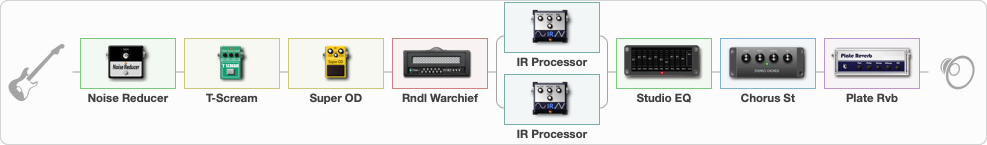# KH BLACK ALBUM

Discussion in 'ToneLib-GFX presets' started by grigoriy0192873, Mar 20, 2023.

1. ### grigoriy0192873Active Member

KH BLACK ALBUM

Preset name: KH BLACK ALBUM

Effects chain:Effect: "Noise Reducer" (Dynamics / Filter), active - "yes"
{
"Sens" = 70
"Mode" = Hard
}

Effect: "T-Scream" (Overdrive / Distortion), active - "yes"
{
"Drive" = 10
"Tone" = 40
"Level" = 10
}

Effect: "Super OD" (Overdrive / Distortion), active - "yes"
{
"Drive" = 40
"Tone" = 40
"Level" = 30
}

Effect: "Rndl Warchief" (Amp simulators), active - "yes"
{
"Gain" = 50
"Bass" = 50
"Middle" = 50
"Treble" = 50
"Presence" = 50
"Master" = 50
"Level (dB)" = 0
}

Effect: "Splitter" (Dynamics / Filter)
{
"A-Bypass" = Off
"A-Pan" = -75
"A-Level" = 55
"B-Bypass" = Off
"B-Pan" = 75
"B-Level" = 55
"Width" = 0

'A' branch:
{

Effect: "IR Processor" (Cabinets), active - "yes"
{
"Low Cut (Hz)" = 0
"Hi Cut (kHz)" = 20.0
"Mix" = 100
"Level (dB)" = 0
}
}
'B' branch:
{

Effect: "IR Processor" (Cabinets), active - "yes"
{
"Low Cut (Hz)" = 0
"Hi Cut (kHz)" = 20.0
"Mix" = 100
"Level (dB)" = 0
}
}
}

Effect: "Studio EQ" (Dynamics / Filter), active - "yes"
{
"31 Hz" = -4
"62 Hz" = -5
"125 Hz" = -6
"250 Hz" = -7
"500 Hz" = -9
"1 kHz" = -8
"2 kHz" = -8
"4 kHz" = -8
"8 kHz" = -8
"16 kHz" = 10
"Level (dB)" = 7
}

Effect: "Chorus St" (Modulation / Sfx), active - "yes"
{
"Speed" = 2.0
"Depth" = 25
"Center" = 10.0
"Mix" = 25
}

Effect: "Plate Rvb" (Reverberation), active - "yes"
{
"Time" = 2.0
"PreDelay" = 20
"LoDamp" = 100
"HiDamp" = 100
"Mix" = 10
}

Note: You will need to download and install the ToneLib-GFX software to use the preset.

File size:
5.3 KB
Views:
1,734
File size:
5.3 KB
Views:
8
File size:
5.3 KB
Views:
7